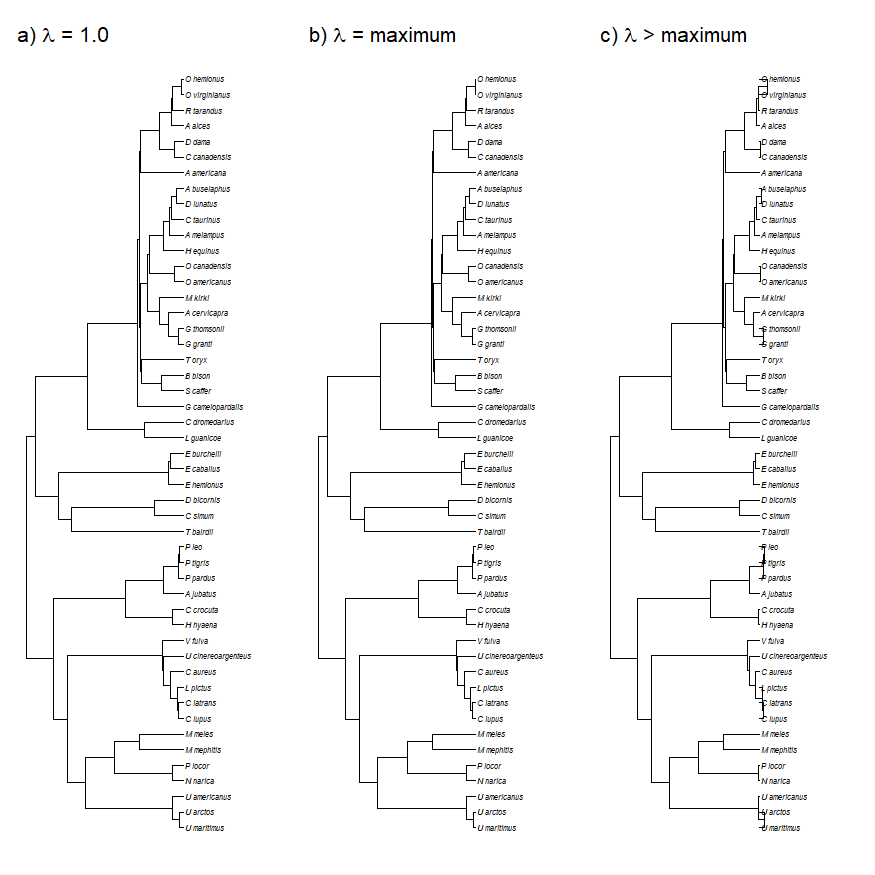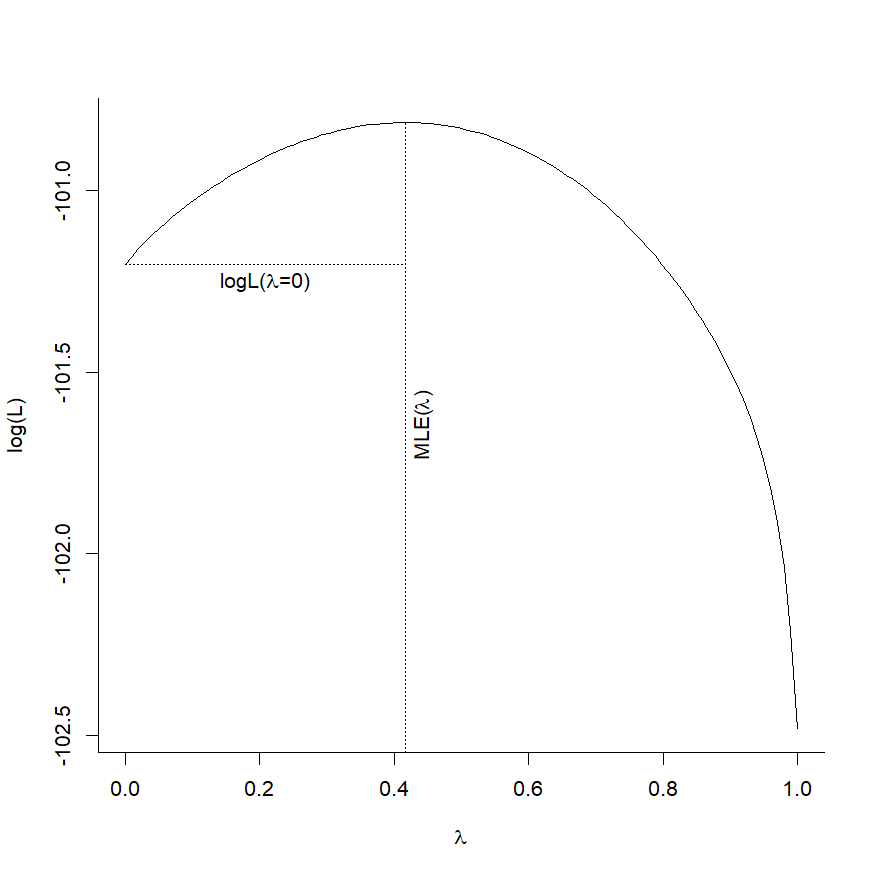## Friday, December 13, 2019

### The maximum value of Pagel's λ (and other tips about optimizing λ using likelihood)

Yesterday, I received an email from a phytools user with the following question:

“I've run across a problem entering starting values for phylosig to estimate Pagel's $$\lambda$$. Could you tell me what the function maxLambda( ) does? Could you also give me a pointer about how to enter starting values for $$\lambda$$ estimation? I'm having some problems with convergence and I'd like to find out if there are any 'attractors' among a set of starting values.”

I'll try to answer both of these questions, but to do so I will use some new features of phytools, so users following along should probably first update phytools from GitHub using devtools.

library(phytools)
packageVersion("phytools")

##  '0.7.11'


I'm also going to use the files mammalHR.phy & mammalHR.csv.

First off, what does the (internal) function maxLambda do? This one's pretty simple. maxLambda compute largest value of $$\lambda$$ for which the correlation matrix implied by the tree is not exactly singular.

What does that mean in simple terms? Well, remember that $$\lambda$$ also corresponds to a tree transformation in which internal branches are multiplied by $$\lambda$$, while terminal edges are adjusted to maintain a constant total height above the root for each tip. maxLambda gives us the largest possible value of $$\lambda$$ such that all the terminal edges are non-zero and thus such that the implied correlation between all pairs of species are < 1. In addition, $$\lambda$$ > than this value implies negative terminal edge lengths.

Let's see what this means using our mammal tree:

mammal.tree<-read.tree("mammalHR.phy")
par(mfrow=c(1,3))
plotTree(mammal.tree,fsize=0.6,ftype="i",
lwd=1,mar=c(1.1,1.1,3.1,1.1))
mtext(expression(paste("a) ",lambda," = 1.0")),line=0,
max.lambda<-phytools:::maxLambda(mammal.tree)
max.lambda

##  1.014493

plotTree(phytools:::lambdaTree(mammal.tree,max.lambda),
fsize=0.6,ftype="i",lwd=1,mar=c(1.1,1.1,3.1,1.1))
mtext(expression(paste("b) ",lambda," = maximum")),line=0,
plotTree(phytools:::lambdaTree(mammal.tree,max.lambda+0.05),
fsize=0.6,ftype="i",lwd=1,mar=c(1.1,1.1,3.1,1.1))
mtext(expression(paste("c) ",lambda," > maximum")),line=0,For the second question, let's load some data for a phenotypic trait - mammal home range size. (I've chosen this trait because I know it has an intermediate value of $$\lambda$$.)

mammal.data<-read.csv("mammalHR.csv",
row.names=1)
homeRange<-setNames(log(mammal.data$homeRange), rownames(mammal.data)) homeRange  ## U_maritimus U_arctos U_americanus N_narica ## 4.75013596 4.41642806 4.03953633 0.04879016 ## P_locor M_mephitis M_meles C_lupus ## 0.13102826 0.91629073 -0.13926207 5.31222027 ## C_latrans L_pictus C_aureus U_cinereoargenteus ## 3.80666249 5.07517382 2.20827441 0.09531018 ## V_fulva H_hyaena C_crocuta A_jubatus ## 1.35325451 5.02912988 3.21887583 4.12874599 ## P_pardus P_tigris P_leo T_bairdii ## 3.14415228 4.24276457 5.46383181 0.69314718 ## C_simum D_bicornis E_hemionus E_caballus ## 1.89461685 2.74727091 3.55534806 3.11351531 ## E_burchelli L_guanicoe C_dromedarius G_camelopardalis ## 5.10594547 -0.69314718 4.60517019 4.43793427 ## S_caffer B_bison T_oryx G_granti ## 4.92725368 4.89034913 4.47163879 2.99573227 ## G_thomsonii A_cervicapra M_kirki O_americanus ## 1.66770682 1.87180218 -3.14655516 3.12456515 ## O_canadensis H_equinus A_melampus C_taurinus ## 2.66235524 4.38202663 1.33500107 4.31748811 ## D_lunatus A_buselaphus A_americana C_canadensis ## 0.78845736 1.60943791 2.30258509 2.55955019 ## D_dama A_alces R_tarandus O_virginianus ## 0.26236426 2.77881927 3.40119738 0.67294447 ## O_hemionus ## 1.04731899  We can estimate $$\lambda$$ as follows: hrangeLambda<-phylosig(mammal.tree, homeRange,method="lambda", test=TRUE) hrangeLambda  ## ## Phylogenetic signal lambda : 0.415998 ## logL(lambda) : -100.813 ## LR(lambda=0) : 0.783684 ## P-value (based on LR test) : 0.376017  It's also now possible to plot the likelihood surface for $$\lambda$$ - which by default computes the surface between 0 & 1 or the MLE of $$\lambda$$ (whichever is higher). plot(hrangeLambda)There is no way to specify a starting value for the optimizer in phylosig since (absent sampling error in our trait) R is doing a univariate optimization on the interval (0,maxLambda); however, we can easily compute the likelihood of any particular value of $$\lambda$$ as follows: hrangeLambda$lik(0)

##  -101.2049

hrangeLambda$lik(0.5)  ##  -100.8297  hrangeLambda$lik(0.7)

##  -101.0179


If we do have sampling error, then optimization is multidimensional (because we have to simultaneously optimize $$\lambda$$ and $$\sigma^2$$) & it is consequently imaginable that the starting value can affect convergence.

Here is a small demo of how to try different initial values for optimization using optim( ). (Note that I could have a different numerical optimization function, such as nlm( ), and it would work more or less the same.)

## imagine some standard errors for each species:
se<-homeRange
se[]<-0.5
se

##        U_maritimus           U_arctos       U_americanus           N_narica
##                0.5                0.5                0.5                0.5
##            P_locor         M_mephitis            M_meles            C_lupus
##                0.5                0.5                0.5                0.5
##          C_latrans           L_pictus           C_aureus U_cinereoargenteus
##                0.5                0.5                0.5                0.5
##            V_fulva           H_hyaena          C_crocuta          A_jubatus
##                0.5                0.5                0.5                0.5
##           P_pardus           P_tigris              P_leo          T_bairdii
##                0.5                0.5                0.5                0.5
##            C_simum         D_bicornis         E_hemionus         E_caballus
##                0.5                0.5                0.5                0.5
##        E_burchelli         L_guanicoe      C_dromedarius   G_camelopardalis
##                0.5                0.5                0.5                0.5
##           S_caffer            B_bison             T_oryx           G_granti
##                0.5                0.5                0.5                0.5
##        G_thomsonii       A_cervicapra            M_kirki       O_americanus
##                0.5                0.5                0.5                0.5
##                0.5                0.5                0.5                0.5
##                0.5                0.5                0.5                0.5
##             D_dama            A_alces         R_tarandus      O_virginianus
##                0.5                0.5                0.5                0.5
##         O_hemionus
##                0.5

hrangeLambda.se<-phylosig(mammal.tree,
homeRange,se=se,method="lambda")
hrangeLambda.se

##
## Phylogenetic signal lambda : 0.441654
## logL(lambda) : -100.813
## MLE(sig2) : 0.0595599

## create temporary likelihood function
LIK<-function(par) hrangeLambda.se$lik(par,par) ## optimize using starting values 0.5 & 0.1 fit1<-optim(c(0.5,0.1),LIK,method="L-BFGS-B", lower=c(0,0),upper=c(1,Inf), control=list(fnscale=-1)) fit1  ##$par
##  0.44160308 0.05955602
##
## $value ##  -100.8131 ## ##$counts
##       49       49
##
## $convergence ##  0 ## ##$message
##  "CONVERGENCE: REL_REDUCTION_OF_F <= FACTR*EPSMCH"

## optimize using starting values of 1.0 & 10
fit2<-optim(c(1,10),LIK,method="L-BFGS-B",
lower=c(0,0),upper=c(1,Inf),
control=list(fnscale=-1))
fit2

## $par ##  0.4416549 0.0595599 ## ##$value
##  -100.8131
##
## $counts ## function gradient ## 33 33 ## ##$convergence
##  0
##
## $message ##  "CONVERGENCE: REL_REDUCTION_OF_F <= FACTR*EPSMCH"  ## optimize using starting values of 0.0 & 100 fit3<-optim(c(0,100),LIK,method="L-BFGS-B", lower=c(0,0),upper=c(1,Inf), control=list(fnscale=-1)) fit3  ##$par
##  0.44163973 0.05955861
##
## $value ##  -100.8131 ## ##$counts
## $convergence ##  0 ## ##$message